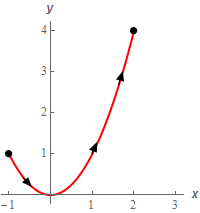Paul's Online Notes
Home / Calculus III / Line Integrals / Line Integrals - Part I
Show Mobile Notice Show All Notes Hide All Notes
Mobile Notice
You appear to be on a device with a "narrow" screen width (i.e. you are probably on a mobile phone). Due to the nature of the mathematics on this site it is best views in landscape mode. If your device is not in landscape mode many of the equations will run off the side of your device (should be able to scroll to see them) and some of the menu items will be cut off due to the narrow screen width.

### Section 16.2 : Line Integrals - Part I

3. Evaluate $$\displaystyle \int\limits_{C}{{6x\,ds}}$$ where $$C$$ is the portion of $$y = {x^2}$$ from $$x = - 1$$ to $$x = 2$$. The direction of $$C$$ is in the direction of increasing $$x$$.

Show All Steps Hide All Steps

Start Solution

Here is a quick sketch of $$C$$ with the direction specified in the problem statement shown.Show Step 2

In this case we can just use the equation of the curve for the parameterization because the specified direction is going in the direction of increasing $$x$$ which will give us integral limits from smaller value to larger value as needed. Here is a parameterization for this curve.

$\vec r\left( t \right) = \left\langle {t,{t^2}} \right\rangle \,\,\,\,\,\,\,\, - 1 \le t \le 2$

We could also break this up into parameter form as follows.

$\begin{array}{*{20}{l}}{x = t}\\{y = {t^2}}\end{array}\hspace{0.25in} - 1 \le t \le 2$

Either form of the parameterization will work for the problem but we’ll use the vector form for the rest of this problem.

Show Step 3

We’ll need the magnitude of the derivative of the parameterization so let’s get that.

$\vec r'\left( t \right) = \left\langle {1,2t} \right\rangle \hspace{0.25in}\hspace{0.25in}\left\| {\vec r'\left( t \right)} \right\| = \sqrt {{{\left( 1 \right)}^2} + {{\left( {2t} \right)}^2}} = \sqrt {1 + 4{t^2}}$

We’ll also need the integrand “evaluated” at the parameterization. Recall all this means is we replace the $$x$$/$$y$$ in the integrand with the $$x$$/$$y$$ from parameterization. Here is the integrand evaluated at the parameterization.

$6x = 6t$ Show Step 4

The line integral is then,

$\int\limits_{C}{{6x\,ds}} = \int_{{ - 1}}^{2}{{6t\sqrt {1 + 4{t^2}} \,dt}} = \left. {\frac{1}{2}{{\left( {1 + 4{t^2}} \right)}^{\frac{3}{2}}}} \right|_{ - 1}^2 = \require{bbox} \bbox[2pt,border:1px solid black]{{\frac{1}{2}\left( {{{17}^{\frac{3}{2}}} - {5^{\frac{3}{2}}}} \right)}}$